Courses

# Multiple Correct MCQ Of Solid State & Surface Chemistry, Past Year Questions, JEE Advance, Class 12, Chemistry

## 8 Questions MCQ Test Class 12 Chemistry 35 Years JEE Mains &Advance Past yr Paper | Multiple Correct MCQ Of Solid State & Surface Chemistry, Past Year Questions, JEE Advance, Class 12, Chemistry

Description
This mock test of Multiple Correct MCQ Of Solid State & Surface Chemistry, Past Year Questions, JEE Advance, Class 12, Chemistry for JEE helps you for every JEE entrance exam. This contains 8 Multiple Choice Questions for JEE Multiple Correct MCQ Of Solid State & Surface Chemistry, Past Year Questions, JEE Advance, Class 12, Chemistry (mcq) to study with solutions a complete question bank. The solved questions answers in this Multiple Correct MCQ Of Solid State & Surface Chemistry, Past Year Questions, JEE Advance, Class 12, Chemistry quiz give you a good mix of easy questions and tough questions. JEE students definitely take this Multiple Correct MCQ Of Solid State & Surface Chemistry, Past Year Questions, JEE Advance, Class 12, Chemistry exercise for a better result in the exam. You can find other Multiple Correct MCQ Of Solid State & Surface Chemistry, Past Year Questions, JEE Advance, Class 12, Chemistry extra questions, long questions & short questions for JEE on EduRev as well by searching above.
*Multiple options can be correct
QUESTION: 1

### Which of the following statement(s) is (are) correct?(1998 - 2 Marks)

Solution:

The crystals of CsCl has body - centred cubic unit cell. Hence, each ion in this structure has coordination number of eight.

NOTE : In case of crystals of NaCl two
interpenetrating face-centred cubic lattices are
present, one of these is composed entirely of Na+ ions and the other of Cl ions. Each Na+ ion is located half way between two Cl ions and viceversa. A unit cell of NaCl crystal has Cl ions at the corners as well as at the face centres and Na+ ions are located in
octahedral voids. On each edge of cubic unit cell, there are two Cl ions and one Na+ ions. Hence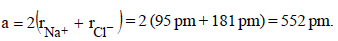*Multiple options can be correct
QUESTION: 2

### The correct statement(s) regarding defects in solids is (are)           (2009S)

Solution:

(b,c)
Frenkel defect is a dislocation effect, observed when the size of the cation and anion differ largely. F-center is created when an anion is lost from the lattice and vacancy is filled by trapping of an electron. Schottky defect changes the density of a crystalline solid.

*Multiple options can be correct
QUESTION: 3

### The correct statement(s) pertaining to the adsorption of agas on a solid surface is (are)        (2011)

Solution:

(a, b, d)
(a) ΔH is negative for adsorption
(b) Fact based
(d) Chemical bonds are stronger than vander Waal’s forces, so chemical adsorption is more exothermic

*Multiple options can be correct
QUESTION: 4

Choose the correct reason(s) for the stability of the lyophobiccolloidal particles. (2012)

Solution:
*Multiple options can be correct
QUESTION: 5

The given graphs/data I, II, III and IV represent general trends observed for different physisorption and chemisorption processes under mild conditions of temperature and presure.

Which of the following choice(s) about I, II, III and IV is (are) correct ?      (2012)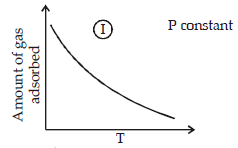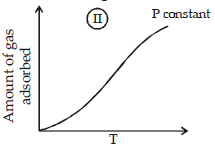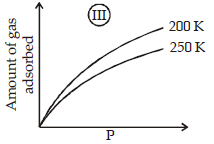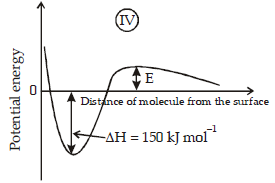Solution:

(a, c)
Graph (I) and (III) represent physisorption because, in
physisorption, the amount of adsorption decreases with the increase of temperature and increases with
the increase of pressure.
Graph (II) represent chemisorption, because in chemisorption amount of adsorption increases with the
increase of temperature. Graph (IV) is showing the formation of a chemical bond, hence chemisorption.

*Multiple options can be correct
QUESTION: 6

If the unit cell of a mineral has cubic close packed (ccp) array of oxygen atoms with m fraction of octahedral holes occupied by aluminium ions and n fraction of tetrahedral holes occupied by magnesium ions, m and n, respectively, are              (JEE Adv. 2015)

Solution:

In ccp, O2– ions are 4.
Hence total negative charge = –8
Let Al3+ ions be x, and Mg2+ ions be y.
Total positive charge = 3x + 2y
⇒ 3x + 2y = 8
This relation is satisfied only by x = 2 and y = 1.
Hence number of Al3+ = 2.
and number of Mg2+ = 1.
⇒ n = fraction of octahedral holes occupied by Al3+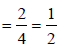and m = fraction of tetrahedral holes occupied by Mg2+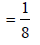*Multiple options can be correct
QUESTION: 7

When O2 is adsorbed on a metallic surface, electron transferoccurs from the metal to O2. The true statement(s) regardingthis adsorption is(are) (JEE Adv. 2015)

Solution:

Reaction on metal surface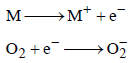This is an example of chemisorption

*Multiple options can be correct
QUESTION: 8

The CORRECT statement(s) for cubic close packed (ccp)three dimensional structure is (are) (JEE Adv. 2016)

Solution:

CCP is ABC ABC …… type packing
(A) In topmost layer, each atom is in contact with 6 atoms in same layer and 3 atoms below this layer

(B) Packing fraction =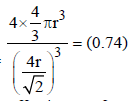(C) Each FCC unit has effective no of atoms = 4
Octahedral void = 4
Tetrahedral void = 8
(D) 4r = a√2